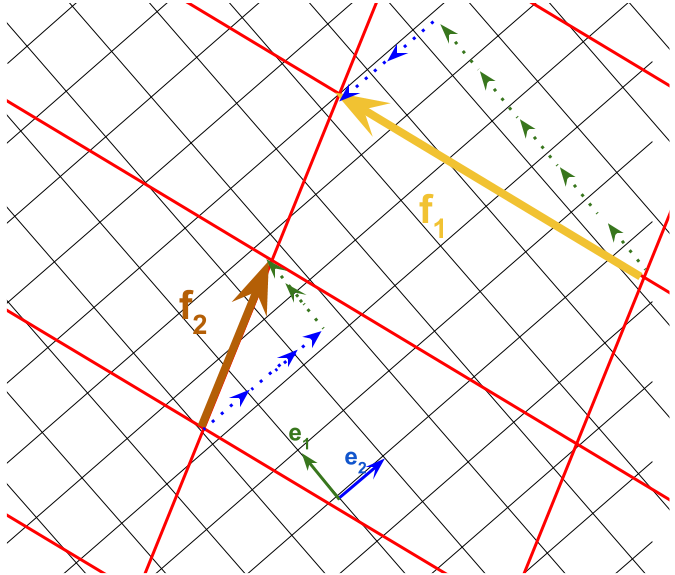### $$\bf Ax=b$$ IS A CHANGE OF BASIS:

We can consider $$A$$ as transformation $$A: \mathbb R^n \rightarrow \mathbb R^m$$ for an $$m \times n$$ matrix.

If we have a basis, $$B=\{\vec v_1, \vec v_2, \dots, v_k\}$$ the coordinates of a vector $$\vec a$$ with respect to this basis can be expressed as:

$$\large [\vec a]_B=\begin{bmatrix}c_1\\c_2\\\vdots\\c_k\end{bmatrix}$$

Equivalently,

$$\large c_1\vec v_1 + c_2\vec v_2+\dots+c_k \vec v_k=\underset{\color{blue}{\text{change of basis matrix 'C'}}}{\underbrace{\color{blue}{\begin{bmatrix}\vert & \vert & &\vert \\v_1&v_2&\dots&v_k\\\vert&\vert&&\vert\end{bmatrix}}}}\begin{bmatrix}c_1\\c_2\\\vdots\\c_k\end{bmatrix}$$

So

$\Large\bbox[yellow, 10px]{ \text{C}\quad [\vec a]_B \quad=\quad [\vec a]_{\text{standard}}}$

The change of basis matrix is the matrix with the basis vectors as columns.

Similarly we can solve for the coordinates of a vector with respect to a basis: If we know that the vector $$\vec d = \begin{bmatrix}8\\-6\\2\end{bmatrix}$$ is in the span of the $$\mathbb R^2$$ basis $$\vec v_1 = \begin{bmatrix}1\\2\\3\end{bmatrix}$$ and $$\vec v_2 = \begin{bmatrix}1\\0\\1\end{bmatrix}$$, we can solve $$\text{C}\,\color{red}{ [\vec d]_B}=\vec d,$$ as a system of linear equations (expanded matrix).

If $$\text{C}$$ is invertible (i.e. it is square and with linearly independent column vectors - a given in a basis), the span of $$\text{C}$$ is $$\mathbb R^n$$, and:

$\large \text{C}^{-1} \quad \text{C}\quad [\vec a]_B= \text{C}^{-1} \quad\vec a$

and hence,

$\bbox[yellow, 10px]{\Large[\vec a]_B\quad= \quad\text{C}^{-1} \quad[\vec a]_{\text{standard}}}$

In general,

$\bbox[yellow, 10px]{\Large[\vec a]_{\text{new coord.}}\quad= \quad\text{C}^{-1} \quad[\vec a]_{\text{old coord}}}\tag{1}$

$$\large \bf A\vec x$$ is a linear transformation (transformation = mapping or function operating on vectors expressed as $$T(\vec x)$$) of $$\vec x$$ with $$\vec x$$ expressed in the standard basis: $$T(\vec x) = A\vec x.$$ But there are multiple different bases. The mapping afforded by the transformation on $$[\vec x]_B$$ will be $$[T(\vec x)]_B.$$ We can find a matrix $$\text{D}$$, such that $[T(\vec x)]_B= \text{D} [\vec x]_B\tag{2}$

$$\text{D}$$ is the transformation matrix for $$T$$ with respect to $$B$$.

Now, $$T(\vec x) = A\vec x$$, and replacing in Eq.2:

$[T(\vec x)]_B=[A\vec x]_B =\text{D} [\vec x]_B$

From Eq.1, $$[A\vec x]_B$$ can be expressed as:

$[A\vec x]_B= \text{C}^{-1} A\vec x$

at the same time $$\vec x = \text{C}[\vec x]_B.$$ Therefore,

$\Large\bbox[yellow, 10px]{\text{D}\quad [\vec x]_B\quad=\quad [A\vec x]_B \quad= \quad \text{C}^{-1}\quad A\quad \text{C}\quad[\vec x]_B}$

We can also see that

$\text{D}\quad\text{C}^{-1}=\text{C}^{-1}\quad A \quad \text{C}\quad\text{C}^{-1}$

or

$\text{C}\quad\text{D}\quad\text{C}^{-1}=\text{C}\quad\text{C}^{-1}\quad A\quad \text{C}\quad\text{C}^{-1}$

Hence,

$\Large A = \text{C D C}^{-1}$

Summary diagram:

$$\require{AMScd}$$ $\begin{CD} \text{STD. COORD: }\vec x @>\text{A}>> T(\vec x)\\ @V V \text{C inv} V @VV \text{C inv} V\\ \text{COORD wrt B: }[\vec x]_B @>>\text{D}> T[(\vec x)]_B \end{CD}$

Any point on the plane is expressed differently by Emily $$\vec e=\begin{bmatrix}\bf \color{green}{e_1} \\ \bf \color{blue}{e_2 }\end{bmatrix}$$ compared to Frank $$\vec f=\begin{bmatrix} \bf \color{orange}{f_1} \\ \bf \color{brown}{f_2} \end{bmatrix}$$.

So we can say that Frank’s basis vector $$\vec f_1=\begin{bmatrix}\color{orange}{1_{\text{f}}}\\\color{brown}{0_{\text{f}}}\end{bmatrix}$$ is $$\vec f_1 = 5 \, \color{green}{e_1} - 2 \, \color{blue}{e_2}$$; and Frank’s basis vector $$\vec f_2=\begin{bmatrix}\color{orange}{0_\text{f}}\\\color{brown}{1_\text{f}}\end{bmatrix}$$ is $$\vec f_2 = 1.5 \, \color{green}{e_1} + 2.5 \, \color{blue}{e_2}$$ from Emily’s perspective.Or we can say that the basis vectors in Frank’s system will be seen from Emily’s perspective as:

$\left(\vec f_1\right)_{\vec e}=\begin{bmatrix}\color{green}{5} & \color{green}{1.5} \\ \color{blue}{-2} & \color{blue}{2.5}\end{bmatrix}\,\begin{bmatrix}\color{orange}{1_{\text{f}}}\\\color{brown}{0_{\text{f}}}\end{bmatrix}=\begin{bmatrix}{\color{green}{5}\\\color{blue}{-2}}\end{bmatrix}$

and

$\left(\vec f_2\right)_{\vec e}=\begin{bmatrix}\color{green}{5} & \color{green}{1.5} \\ \color{blue}{-2} & \color{blue}{2.5}\end{bmatrix}\,\begin{bmatrix}\color{orange}{0_{\text{f}}}\\\color{brown}{1_{\text{f}}}\end{bmatrix}=\begin{bmatrix}{\color{green}{1.5}\\\color{blue}{2.5}}\end{bmatrix}$

So in the columns of $$\bf A =\begin{bmatrix}\color{green}{5} & \color{green}{1.5} \\ \color{blue}{-2} & \color{blue}{2.5}\end{bmatrix}$$ we have stored how the basis vectors in Frank’s system are expressed in Emily’s basis:

$\bf A =\begin{bmatrix}\color{green}{5} & \color{green}{1.5} \\ \color{blue}{-2} & \color{blue}{2.5}\end{bmatrix} = \begin{bmatrix}\vert & \vert \\ (\vec f_1)_{\vec e} & (\vec f_2)_{\vec e} \\ \vert & \vert \end{bmatrix}$

If we were to express in Emily’s system some other vector initially given in Frank’s system we would just have to perform the operation:

\begin{align}\begin{bmatrix}\bf y_{1e}\\\bf{y_{2e}}\end{bmatrix}&= \begin{bmatrix}\color{green}{5} & \color{green}{1.5} \\ \color{blue}{-2} & \color{blue}{2.5}\end{bmatrix}\begin{bmatrix}\bf x_{1f}\\\bf x_{2f}\end{bmatrix}\\[2ex] &=\bf x_{1f}\begin{bmatrix}\color{green}{5}\\\color{blue}{-2}\end{bmatrix} + \bf x_{2f}\begin{bmatrix}\color{green}{1.5}\\\color{blue}{2.5}\end{bmatrix} \end{align}

The thing to note is that $$\bf x_{1f}$$ will multiply the first column in the matrix to contribute to $$\bf y$$ (the first basis vector contribution); and $$\bf x_{2f}$$ will multiply the second column vector to contribute to $$\bf y$$ (the second basis vector contribution, expressed in Emily’s language).

Clearly we have just made up the equivalence between vector basis in between systems, and any other matrix $$\bf A$$ would have served the purpose. Therefore we can say that the operation $$\bf Ax = b$$ is the equivalent of a change of basis: $$\begin{bmatrix}{\bf y_{1e} \\ \bf y_{2e}}\end{bmatrix}$$ is $$\begin{bmatrix}{\bf x_{1f} \\ \bf x_{2f}}\end{bmatrix}$$ viewed from Emily’s perspective!

So is the system of equations:

\begin{align} 5{\bf x_{1f}} + 1.5\bf{x_{2f}}&=\bf{y_{1e}}\\ -2{\bf x_{1f}} + 2.5 \bf{x_{2f}} &=\bf{y_{2e}} \end{align}

Can we express Emily’s vectors from Frank’s perspective? Yes:

\begin{align} \frac{5}{31}{\bf y_{1e}} \frac{-3}{31}\bf{y_{2e}}&=\bf{x_{1f}}\\ \frac{4}{31}{\bf y_{1f}} + \frac{10}{31} \bf{y_{2e}} &=\bf{x_{2f}} \end{align}

or

$\begin{bmatrix}\color{green}{5} & \color{green}{1.5} \\ \color{blue}{-2} & \color{blue}{2.5}\end{bmatrix}^{-1}=\begin{bmatrix}\color{orange}{\frac{5}{31}}&\color{orange}{\frac{-3}{31}}\\\color{brown}{\frac{4}{31}}&\color{brown}{\frac{10}{31}}\end{bmatrix}$

So now we can look at the Emily’s coordinates vector $$\begin{bmatrix}\bf y_{1e}\\ \bf y_{1e}\end{bmatrix}$$ in Frank’s coordinates:

$\begin{bmatrix}\color{orange}{\frac{5}{31}}&\color{orange}{\frac{-3}{31}}\\\color{brown}{\frac{4}{31}}&\color{brown}{\frac{10}{31}}\end{bmatrix}\begin{bmatrix}{\bf y_{1e} \\ y_{1e}}\end{bmatrix}=\begin{bmatrix}{\bf x_{1f} \\ \bf x_{2f}}\end{bmatrix}$

Likewise, the basis vectors of Emily with respect to Frank’s are:

$\left(\vec e_1\right)_{\vec f}=\begin{bmatrix}\color{orange}{\frac{5}{31}}&\color{orange}{\frac{-3}{31}}\\\color{brown}{\frac{4}{31}}&\color{brown}{\frac{10}{31}}\end{bmatrix}\,\begin{bmatrix}\color{green}{1_{\text{e}}}\\\color{blue}{0_{\text{e}}}\end{bmatrix}=\begin{bmatrix}{\color{orange}{\frac{5}{31}}\\\color{brown}{\frac{4}{31}}}\end{bmatrix}$

and

$\left(\vec e_2\right)_{\vec f}=\begin{bmatrix}\color{orange}{\frac{5}{31}}&\color{orange}{\frac{-3}{31}}\\\color{brown}{\frac{4}{31}}&\color{brown}{\frac{10}{31}}\end{bmatrix}\,\begin{bmatrix}\color{green}{0_{\text{e}}}\\\color{blue}{1_{\text{e}}}\end{bmatrix}=\begin{bmatrix}{\color{orange}{\frac{-3}{31}}\\\color{brown}{\frac{10}{31}}}\end{bmatrix}$